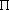## 2.3 RANDOM VARIABLES

Many, if not most, experiments have numerical values associated with different outcomes. In fact, for some experiments the finest-grained outcomes are described directly in terms of numbers. This is the case for the triangleproblem, but not for a simple flip of a coin (in which the two possible outcomes are "heads" or "tails"). But even for the flip of the coin we may wish to associate numerical values with the outcomes, say +\$1 for "heads" and - \$2 for "tails." To facilitate such numerical descriptions of the outcomes of an experiment, we introduce the notion of a random variable.

Definition: Given an experiment with a sample space and a probability assignment over the sample space, a random variable is a function that assigns a numerical value to each finest-grained outcome in the sample space.

Note: While each finest-grained outcome is unique, it is not necessary that each assigned value of the random variable be unique. Thus, two or more finest-grained points may yield the same value of the random variable.

We shall usually denote random variables by capital letters, such as X, Y, or Z. Each of these represents a complete set of correspondences between finest-grained outcomes in the experiment, with their probability assignments, and associated numerical values. Thus, the notation X does not refer to a number such as 2.3 or -but rather to a listing (or, if you like, mapping) which provides a numerical value for the random variable for each point in the sample space. This sample space is assumed to have a probability assignment associated with it. Once an experiment is carried out, each particular outcome yields specific numerical values for the random variables, say X = x, Y = y, and Z = z. In general and whenever convenient, we will use the same letter (but in lowercase form) to indicate a particular experimental value of the random variable. However, it would also be entirely reasonable to say thatX = 2.62, X = a, X = c +/3, or X = any other number or representation for a number.

The set of possible values for a random variable is called its event space. For the purpose of summing probabilities, it is convenient to discuss separately discrete random variables, whose event spaces contain a finite or countably infinite number of values, and continuous random variables, whose event spaces contain a noncountably infinite number of values.